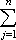#Interactive Real Analysis

Next | Previous | Glossary | Map

## 7.1. Riemann Integral

### Definition 7.1.5: Upper and Lower Sum

Let P = { x0, x1, x2, ..., xn} be a partition of the closed interval [a, b] and f a bounded function defined on that interval. Then:

The upper sum of f with respect to the partition P is defined as:

U(f, P) =cj (xj - xj-1)
where cj is the supremum of f(x) in the interval [xj-1, xj].

The lower sum of f with respect to the partition P is defined as

L(f, P) =dj (xj - xj-1)
where dj is the infimum of f(x) in the interval [xj-1, xj].
Next | Previous | Glossary | Map